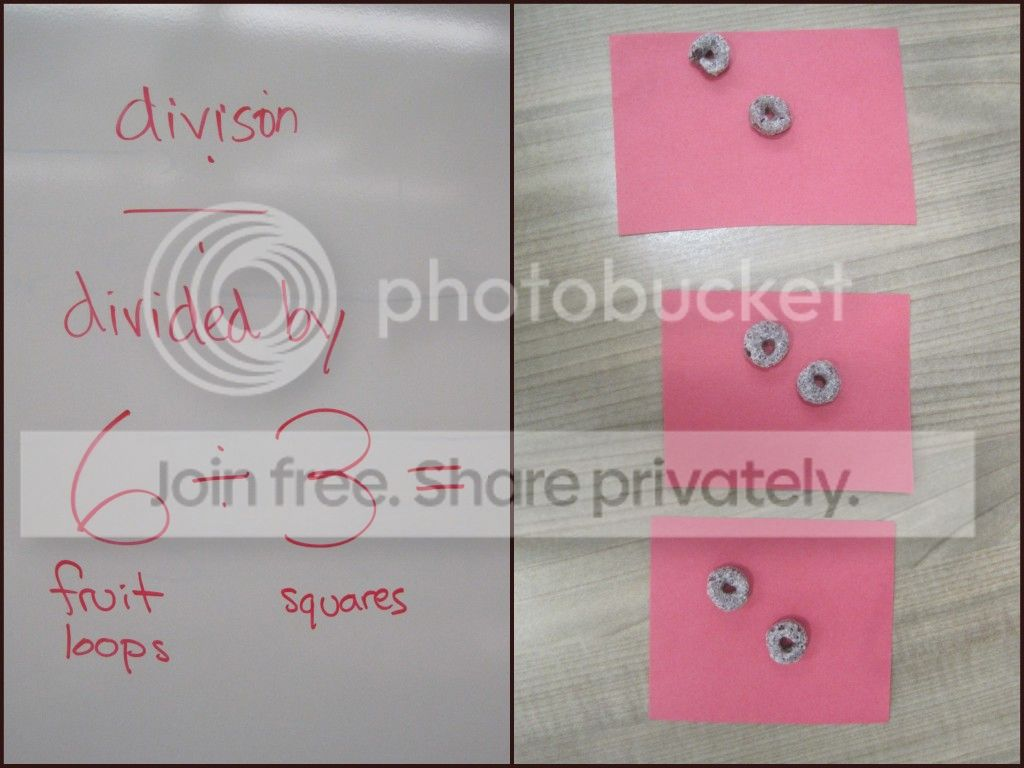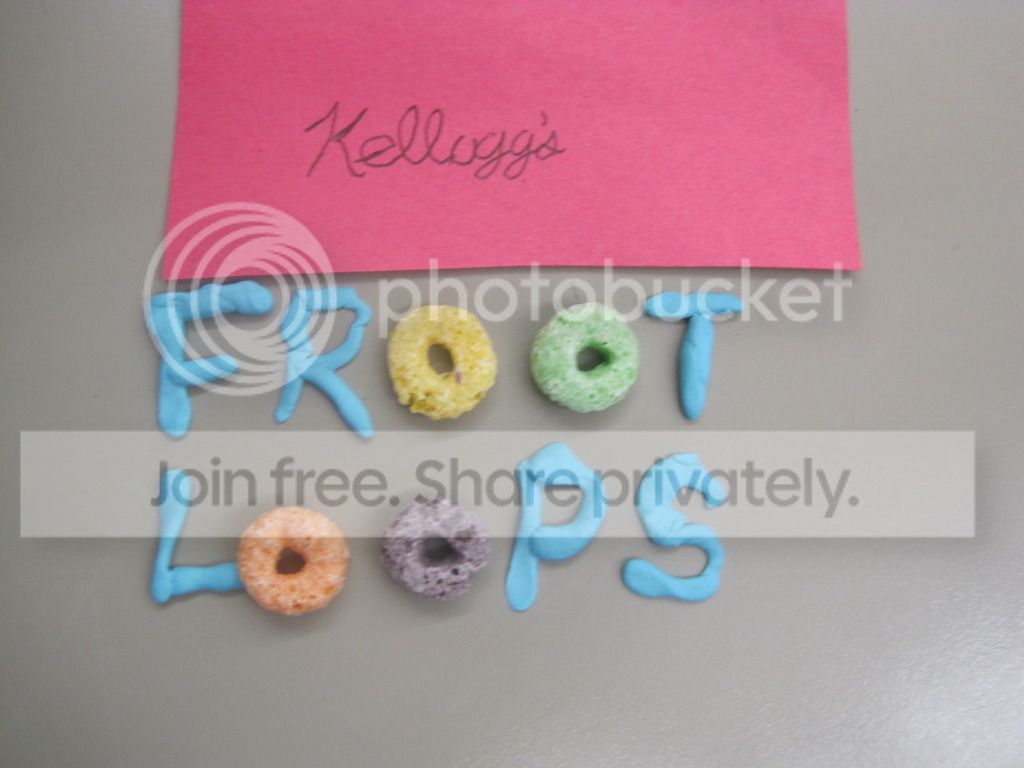### Teaching Multiplication and Division

My students have been BEGGING me to learn multiplication and division. So, I caved. :) I just introduced the concept last week using fruit loops and little construction paper squares. (These were simply the items that were available to me - I would recommend using a mono-colored cereal, some of my students were focused on color sorting the cereal instead of multiplying/dividing)

Hopefully this is pretty self explanatory, but here's some examples.

Division: For a problem like 6 divided by 3, I told my students to first count out 6 fruit loops. Then they were to divide the 6 fruit loops equally between 3 of their squares. So, how many fruit loops are on each square?Multiplication: For a problem like 3 times 3, I would tell my students to put 3 fruit loops on each of the 3 squares. Then, count how many total fruit loops you have on the squares. (Sorry, I don't have a picture of this one)

And one of my students grabbed some sticky tack and I found this on his desk :)I also created a worksheet with some word problems and space to solve them using this type of visual method, and so far it's working great!

1.2.